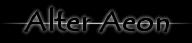alteraeon.com 3000

# Alter Aeon Help Page Search

```
Search For:

Search results for 'calculate'

Keywords are:  'math calculations calculator calculate'

Syntax:
calculate <expression>
calculate <variablename> = <expression>

Note that this code is very rudimentary, and might be buggy.

An expression consists of numbers and operators, for example 3.14*16*16.
There is NO operator precedence.  Parenthesis do work however, so anything
inside of them will be done first.  If you really need things to be
calculated in a specific order, use parenthesis to guarantee it.

If you specify a variable name, the result of the calculation will be put
into the given variable.  For example:

calculate spam = 4+(7*dieroll(3,6))

The result '11' will be put into the variable 'spam'.  You can use the
'variables' command to see which variables you currently have set.

Available functions are:
log(number)              - log base 10 of number
ln(number)               - natural log of number
exp(number)              - e^number
sqrt(number)             - square root of number
rnd(number)              - a random integer between 1 and number
dieroll(rolls,diesize)   - a random dieroll

Available arithmetic operators: * / + -
Available bitwise operators:    & | ^
```